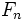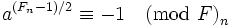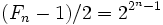# Difference between revisions of "Quadratic nonresidue equals primitive root for Fermat prime"

## Statement

Letbe a nonnegative integer such that the Fermat numberis prime, i.e., is a Fermat prime. Then, ifis a quadratic nonresidue modulo, thenis a primitive root modulo.

## Proof

Given: A Fermat prime, a quadratic nonresiduemodulo.

To prove:is a primitive root modulo.

Proof: We need to show that the order ofmodulois.

By fact (1), ifis a quadratic nonresidue modulo,. Thus, the order ofmodulodoes not divide. On the other hand, the order divides. Thus, the order ofmoduloequals, sois a primitive root modulo.